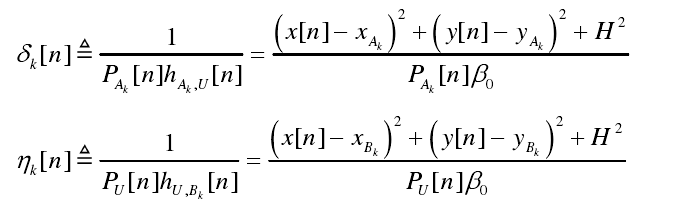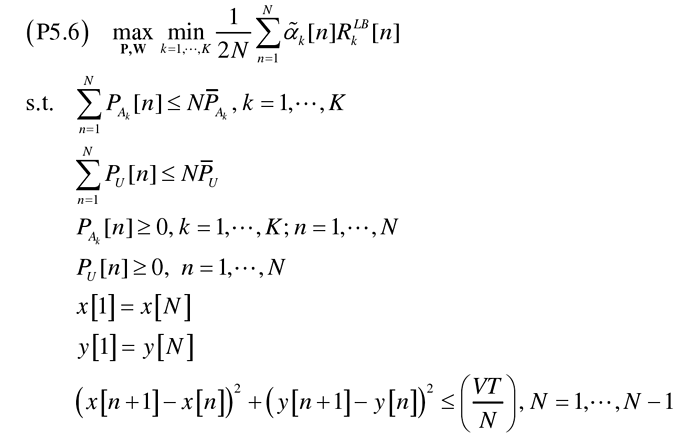# How to express these formulas correctly in CVX?x[n],y[n],Pu[n],Pak[n] are variables to optimize.
for i = 1:K
for j=1:N
deltal(j,i) = prod_inv([inv_pos(((xl(j)-Ax(i)).^2+(yl(j)-Ay(i)).^2+h^2)), Pal(j,i)])/beta0;
end
end
Both these two methods above can not solve my problem.

`quad_over_lin`, with the first argument being a 3 element vector, consisting of one element for each term being squared,should work.

quad_over_lin Sum of squares over linear.
Z=quad_over_lin(X,Y), where X is a vector and Y is a scalar, is equal to
SUM(ABS(X).^2)./Y if Y is positive, and +Inf otherwise. Y must be real.

``````If X is a matrix, quad_over_lin(X,Y) is a row vector containing the values
of quad_over_lin applied to each column. If X is an N-D array, the operation
is applied to the first non-singleton dimension of X.

quad_over_lin(X,Y,DIM) takes the sum along the dimension DIM of X.
A special value of DIM == 0 is accepted here, which is automatically
replaced with DIM == NDIMS(X) + 1. This has the effect of eliminating
the sum; thus quad_over_lin( X, Y, NDIMS(X) + 1 ) = ABS( X ).^2 ./ Y.

In all cases, Y must be compatible in the same sense as ./ with the squared
sum; that is, Y must be a scalar or the same size as SUM(ABS(X).^2,DIM).

Disciplined convex programming information:
quad_over_lin is convex, nonmontonic in X, and nonincreasing in Y.
Thus when used with CVX expressions, X must be convex (or affine)
and Y must be concave (or affine).``````

Thank you for helping me and my problem has been solved. I should just think in another point of view## Excues me, May I ask why this problem is infeasible?U)U@IT9({LT48PHBEM7JJ1315×828 26.4 KBHere is my code: cvx_begin variable xl(N,1) variable yl(N,1) variable Pal(N,K) variable Pul(N,1) expression deltal(N,K) expression yetal(N,K) expression R(N,K) for i = 1:K for j=1:N deltal(j,i) = quad_over_lin([(xl(j)-Ax(i)),(yl(j)-Ay(i)),h],Pal(j,i))/beta0; yetal(j,i) = quad_over_lin([(xl(j)-Bx(i)),(yl(j)-By(i)),h],Pul(j))/beta0; end end R = log2(1+gama)-C.(deltal-delta)-D.(yetal-yeta); maximize min(diag(aR)) subject to sum(Pal)<=NavPa; sum(Pul)<=NavPu; Pal>=0; Pul>=0; xl(1)==xl(N); yl(1)==yl(N); (arrxl).^2+(arryl).^2-(VT/N)^2<=0; cvx_endand here is the cvx information: Calling SDPT3 4.0: 6304 variables, 1997 equality constraints For improved efficiency, SDPT3 is solving the dual problem.

num. of constraints = 1997
dim. of sdp var = 396, num. of sdp blk = 198
dim. of socp var = 5000, num. of socp blk = 1000
dim. of linear var = 710
number of dense column in A = 5
checkdepconstr: AAt is not pos. def.

SDPT3: Infeasible path-following algorithms

## number of iterations = 31 residual of dual infeasibility certificate X = 1.04e-06 reldist to infeas. <= 2.18e-07 Total CPU time (secs) = 6.31 CPU time per iteration = 0.20 termination code = 2 DIMACS: 1.5e-03 0.0e+00 2.0e-03 0.0e+00 -1.0e+00 1.3e+00

Status: Infeasible
Optimal value (cvx_optval): -Inf

Except for section 1, the advice in https://yalmip.github.io/debugginginfeasible also applies to CVX.